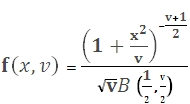# SQL Server Student's T distribution function

STUDENTST

Updated: 9 August 2010

Use STUDENTST to calculate Student’s t-distribution. The formula is:Where
x              is a random variable
v              is the degrees of freedom
Syntax
SELECT [wctStatistics].[wct].[STUDENTST] (
<@X, float,>
,<@Degrees_freedom, float,>
,<@Cumulative, bit,>)
Arguments
@X
is the value supplied to the distribution. @X is an expression of type float or of a type that can be implicitly converted to float
@Degrees_freedom
is a number specifying the degrees of freedom. @Degrees_freedom is an expression of type float or of a type that can be implicitly converted to float
@Cumulative
identifies the distribution calculation as being either the cumulative distribution function or the probability density function.   @Cumulative is an expression of type Boolean or of a type that can be implicitly converted to Boolean.
Return Types
float
Remarks
·         If @Degrees_freedom < 1 the function returns an error
·         If @X < 0 the function returns an error
·         @Degrees_freedom is truncated to zero decimal places
·         Specify the cumulative distribution function by entering 'True' or 1 in @Cumulative
·         Specify the probability density function by entering 'False' or 0 in @Cumulative
Examples
To calculate the probability density function (pdf)
SELECT wct.STUDENTST(1, 2, 'False')

This produces the following result
----------------------
0.192450089729875

(1 row(s) affected)

This may also be entered as
SELECT wct.STUDENTST(1, 2, 0)

To calculate the cumulative distribution function (cdf)
SELECT wct.STUDENTST(1, 2, 'True')

This produces the following result
----------------------
0.788675134594813

(1 row(s) affected)
This may also be entered as
SELECT wct.STUDENTST(1, 2, 1)

### SupportCopyright 2008-2021 Westclintech LLC         Privacy Policy        Terms of Service